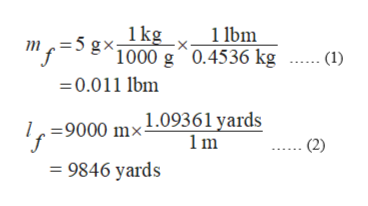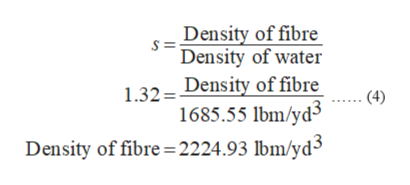# In the textile industry, filament and yarn sizes are reported in denier which is defined as the mass in grams of a length of 9000 meters. IF a synthetic fiber has an average specific gravity of 1.32 and filament of this material has a denier of 5.0, what is the mass per unit length is pounds-mass per yard? What is the cross-sectional area of this fiber in square inches? The specific gravity is defined as: specific gravity=density/(density of water)

Question
68 views

In the textile industry, filament and yarn sizes are reported in denier which is defined as the mass in grams of a length of 9000 meters. IF a synthetic fiber has an average specific gravity of 1.32 and filament of this material has a denier of 5.0, what is the mass per unit length is pounds-mass per yard? What is the cross-sectional area of this fiber in square inches? The specific gravity is defined as: specific gravity=density/(density of water)

check_circle

Step 1

Given data:

Average specific gravity of synthetic fibre (s) = 1.32

Denier of the given filament = 5

It is known that Denier is mass (in grams) of the fibre which is 9000 m (lf) in length. As the Denier of this filament is 5, therefore, the mass of the given fibre (mf) is 5 grams.

To calculate mass per unit length of this fibre the following unit conversions are helpful.

Unit conversion:

1 kg = 1000 g

1 lbm = 0.4536 kg

1 m = 1.09361 yards

The unit conversion of mass of the given fibre (in kg) and that of its length (in yards) is given in equation (1) and (2) respectively.help_outlineImage Transcriptionclose1 lbm 1kg m g1000 g^0.4536 kg .. (1) 0.011 lbm 9000 mx1.09361 yards 1m (2) 9846 yards fullscreen
Step 2

Mass per unit length is calculated using equations (1) and (2) as shown in equation (3).

Step 3

The specific gravity is defined as the ratio of density of component to the density of water at 4 oC,...help_outlineImage TranscriptioncloseDensity of fibre Density of water 1.32 Density of fibre 1685.55 lbm/yd3 Density of fibre 2224.93 lbm/yd3 (4) fullscreen

### Want to see the full answer?

See Solution

#### Want to see this answer and more?

Solutions are written by subject experts who are available 24/7. Questions are typically answered within 1 hour.*

See Solution
*Response times may vary by subject and question.
Tagged in

### Chemical Engineering